# RD Sharma Solutions for Class 8 Maths Chapter 2 - Powers Exercise 2.1

Students can refer and download RD Sharma Solutions for Class 8 Maths Exercise 2.1 Chapter 2, Powers from the links provided below.

Our experts have solved the RD Sharma Solutions to ensure that the students are thorough with their basic concepts and help clear their doubts. Exercise 2.1 is based on the negative integral exponents. Students can download the Solutions for RD Sharma Class 8 Maths Chapter 2 and start practising them offline.

## Download PDF of RD Sharma Solutions for Class 8 Maths Exercise 2.1 Chapter 2 Powers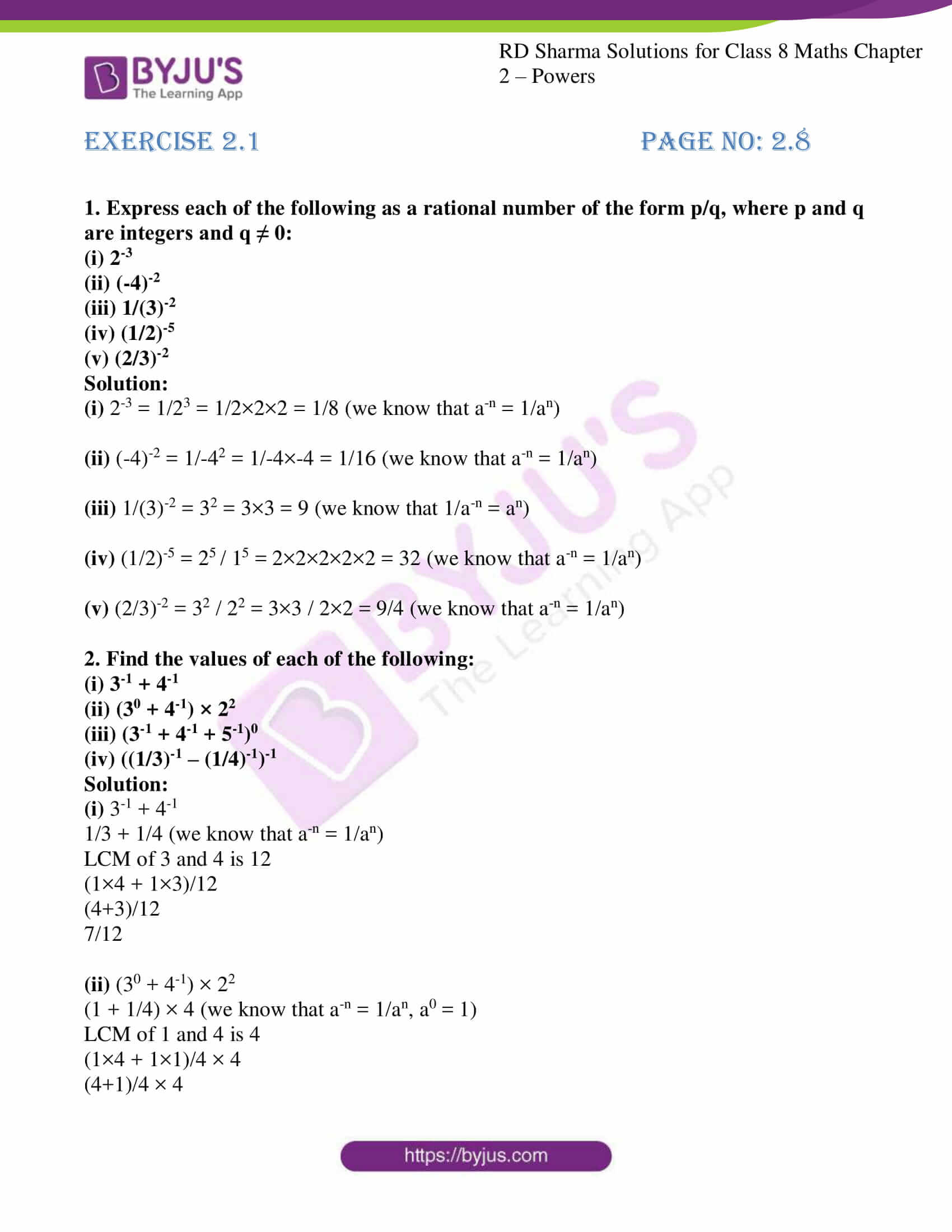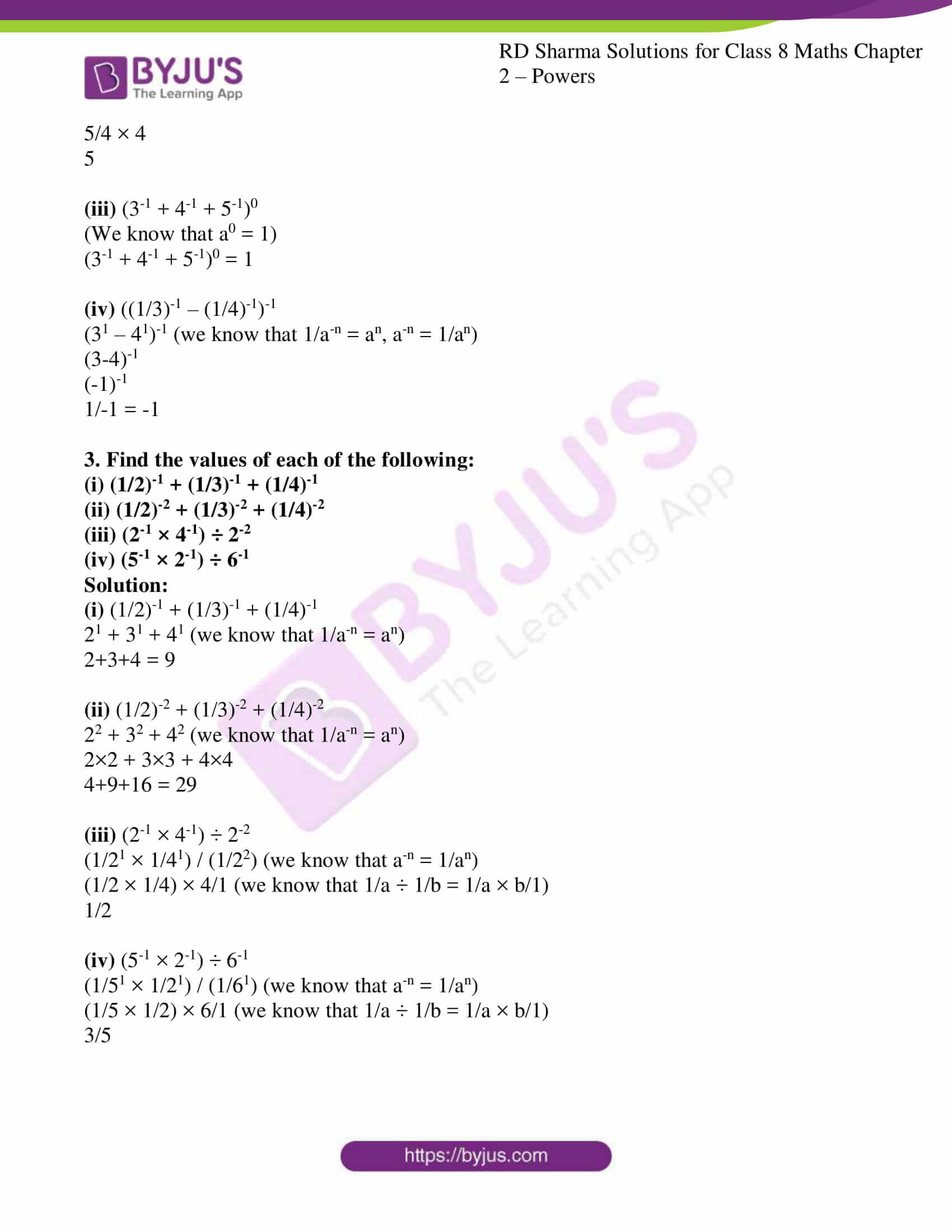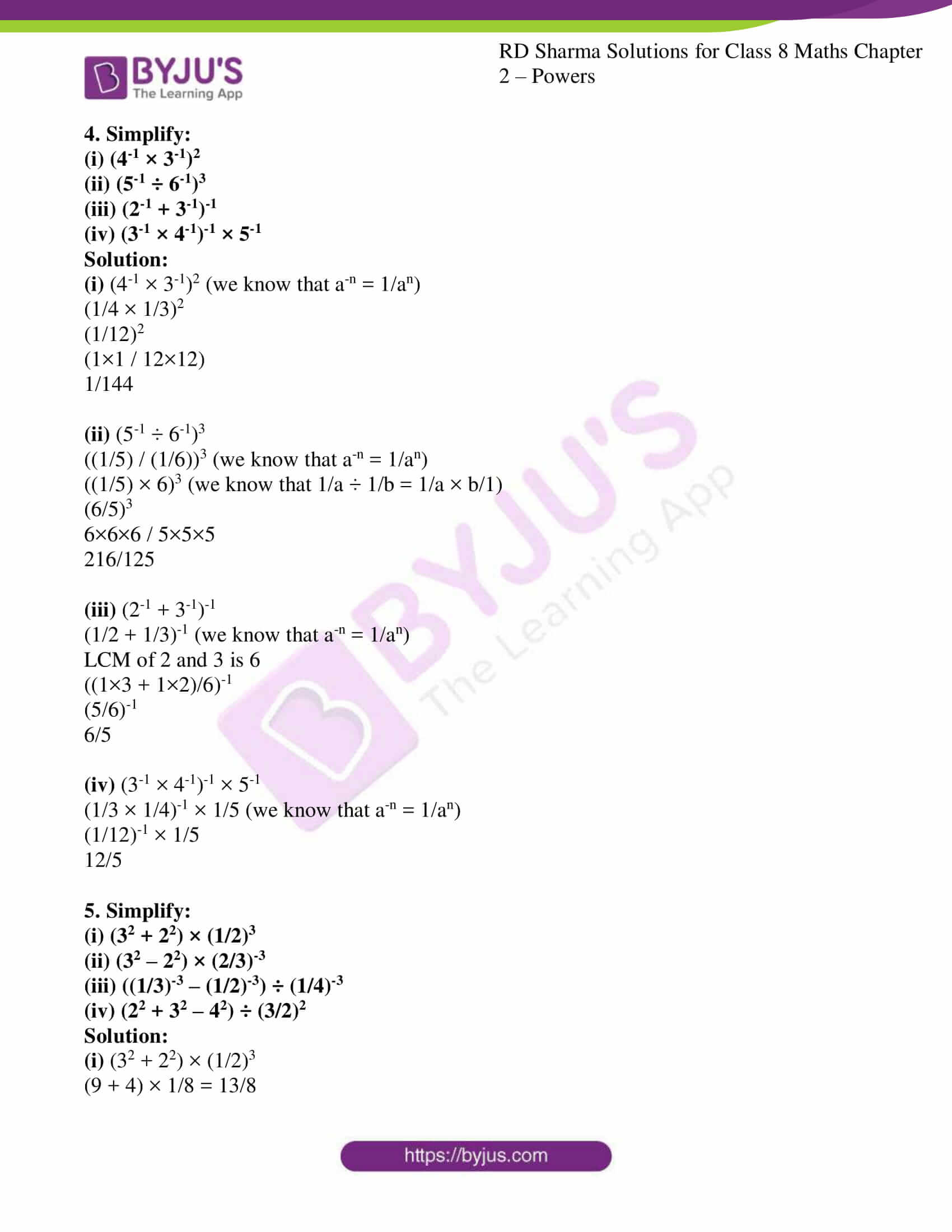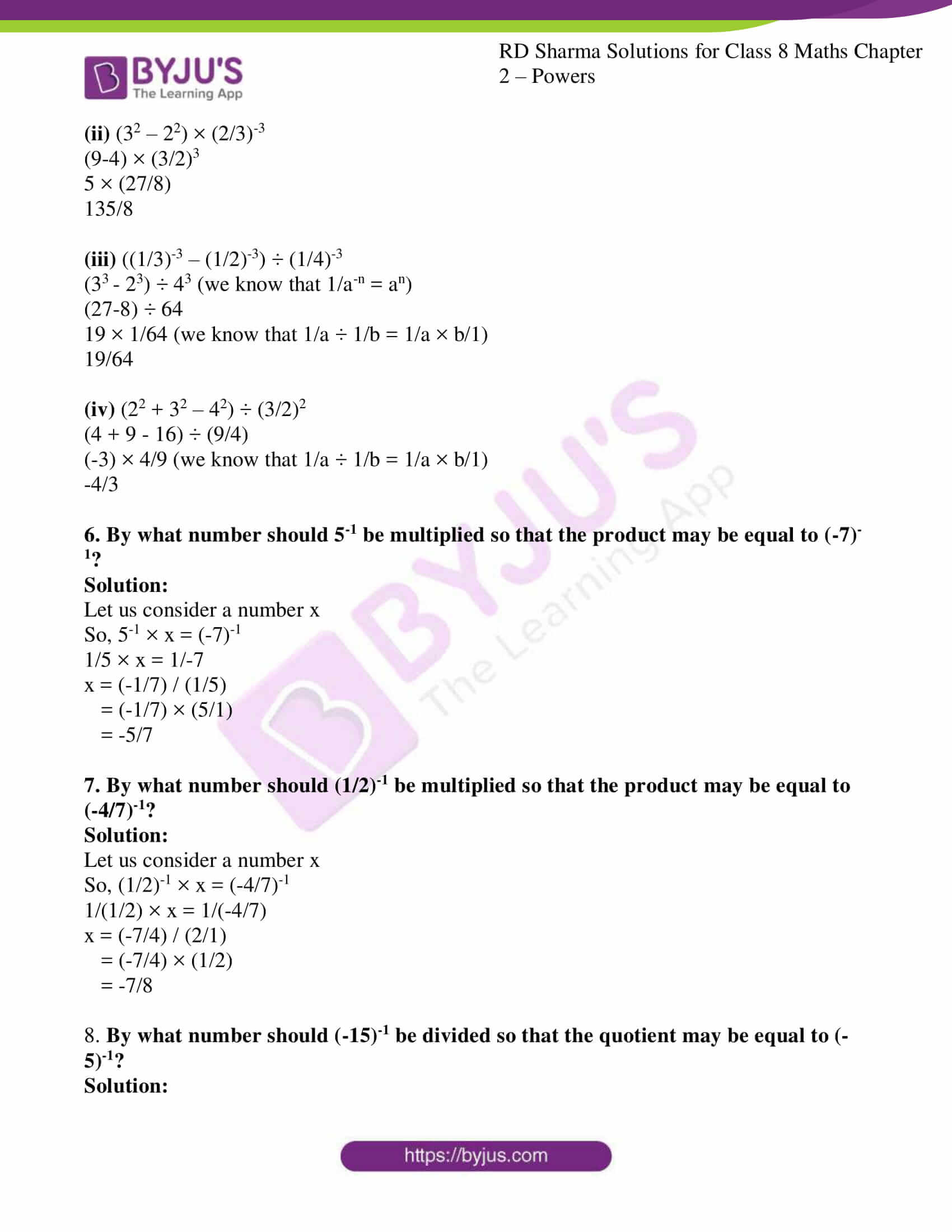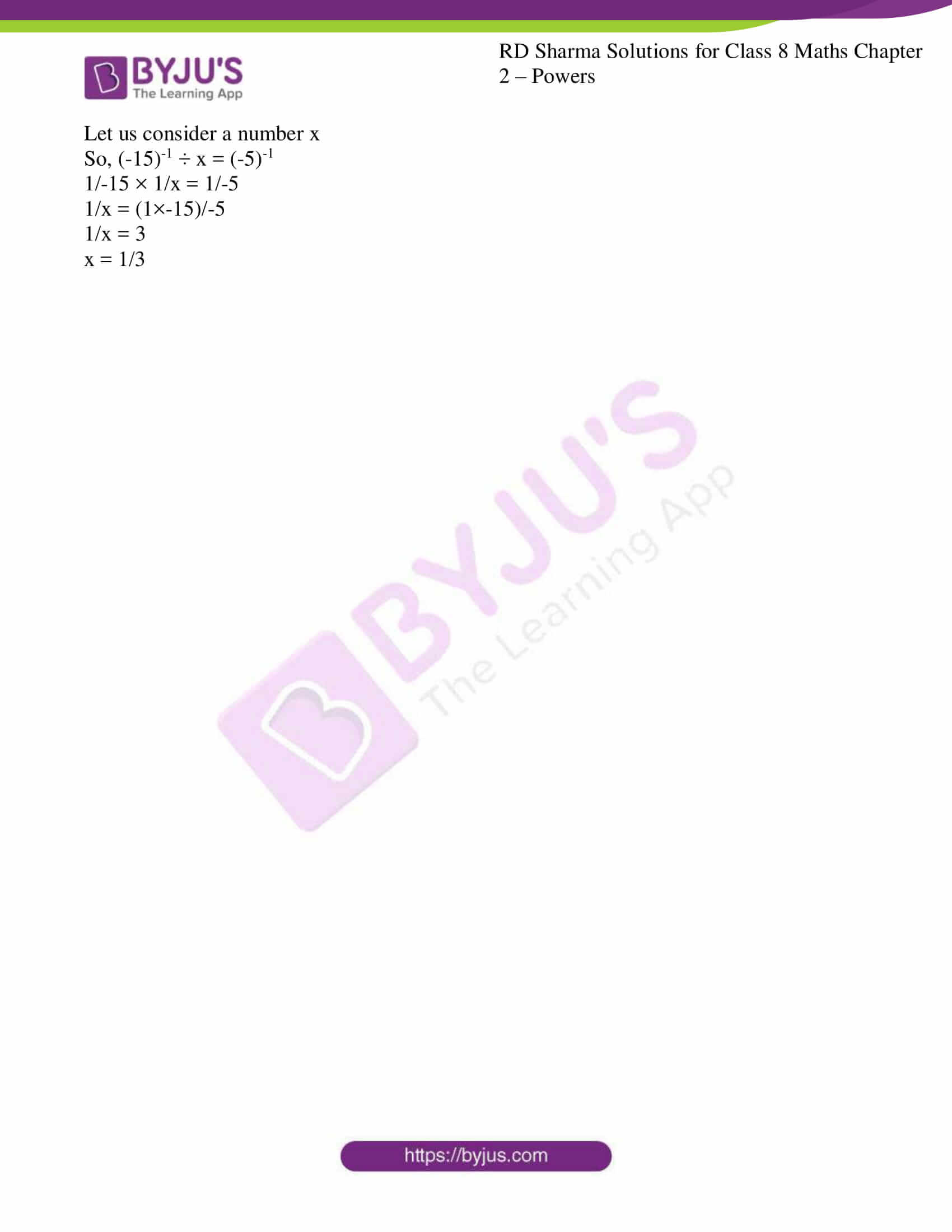### Access Answers to RD Sharma Solutions for Class 8 Maths Exercise 2.1 Chapter 2 Powers

1. Express each of the following as a rational number of the form p/q, where p and q are integers and q ≠ 0:

(i) 2-3

(ii) (-4)-2

(iii) 1/(3)-2

(iv) (1/2)-5

(v) (2/3)-2

Solution:

(i) 2-3 = 1/23 = 1/2×2×2 = 1/8 (we know that a-n = 1/an)

(ii) (-4)-2 = 1/-42 = 1/-4×-4 = 1/16 (we know that a-n = 1/an)

(iii) 1/(3)-2 = 32 = 3×3 = 9 (we know that 1/a-n = an)

(iv) (1/2)-5 = 25 / 15 = 2×2×2×2×2 = 32 (we know that a-n = 1/an)

(v) (2/3)-2 = 32 / 22 = 3×3 / 2×2 = 9/4 (we know that a-n = 1/an)

2. Find the values of each of the following:

(i) 3-1 + 4-1

(ii) (30 + 4-1) × 22

(iii) (3-1 + 4-1 + 5-1)0

(iv) ((1/3)-1 – (1/4)-1)-1

Solution:

(i) 3-1 + 4-1

1/3 + 1/4 (we know that a-n = 1/an)

LCM of 3 and 4 is 12

(1×4 + 1×3)/12

(4+3)/12

7/12

(ii) (30 + 4-1) × 22

(1 + 1/4) × 4 (we know that a-n = 1/an, a0 = 1)

LCM of 1 and 4 is 4

(1×4 + 1×1)/4 × 4

(4+1)/4 × 4

5/4 × 4

5

(iii) (3-1 + 4-1 + 5-1)0

(We know that a0 = 1)

(3-1 + 4-1 + 5-1)0 = 1

(iv) ((1/3)-1 – (1/4)-1)-1

(31 – 41)-1 (we know that 1/a-n = an, a-n = 1/an)

(3-4)-1

(-1)-1

1/-1 = -1

3. Find the values of each of the following:

(i) (1/2)-1 + (1/3)-1 + (1/4)-1

(ii) (1/2)-2 + (1/3)-2 + (1/4)-2

(iii) (2-1 × 4-1) ÷ 2-2

(iv) (5-1 × 2-1) ÷ 6-1

Solution:

(i) (1/2)-1 + (1/3)-1 + (1/4)-1

21 + 31 + 41 (we know that 1/a-n = an)

2+3+4 = 9

(ii) (1/2)-2 + (1/3)-2 + (1/4)-2

22 + 32 + 42 (we know that 1/a-n = an)

2×2 + 3×3 + 4×4

4+9+16 = 29

(iii) (2-1 × 4-1) ÷ 2-2

(1/21 × 1/41) / (1/22) (we know that a-n = 1/an)

(1/2 × 1/4) × 4/1 (we know that 1/a ÷ 1/b = 1/a × b/1)

1/2

(iv) (5-1 × 2-1) ÷ 6-1

(1/51 × 1/21) / (1/61) (we know that a-n = 1/an)

(1/5 × 1/2) × 6/1 (we know that 1/a ÷ 1/b = 1/a × b/1)

3/5

4. Simplify:

(i) (4-1 × 3-1)2

(ii) (5-1 ÷ 6-1)3

(iii) (2-1 + 3-1)-1

(iv) (3-1 × 4-1)-1 × 5-1

Solution:

(i) (4-1 × 3-1)2 (we know that a-n = 1/an)

(1/4 × 1/3)2

(1/12)2

(1×1 / 12×12)

1/144

(ii) (5-1 ÷ 6-1)3

((1/5) / (1/6))3 (we know that a-n = 1/an)

((1/5) × 6)3 (we know that 1/a ÷ 1/b = 1/a × b/1)

(6/5)3

6×6×6 / 5×5×5

216/125

(iii) (2-1 + 3-1)-1

(1/2 + 1/3)-1 (we know that a-n = 1/an)

LCM of 2 and 3 is 6

((1×3 + 1×2)/6)-1

(5/6)-1

6/5

(iv) (3-1 × 4-1)-1 × 5-1

(1/3 × 1/4)-1 × 1/5 (we know that a-n = 1/an)

(1/12)-1 × 1/5

12/5

5. Simplify:

(i) (32 + 22) × (1/2)3

(ii) (32 – 22) × (2/3)-3

(iii) ((1/3)-3 – (1/2)-3) ÷ (1/4)-3

(iv) (22 + 32 – 42) ÷ (3/2)2

Solution:

(i) (32 + 22) × (1/2)3

(9 + 4) × 1/8 = 13/8

(ii) (32 – 22) × (2/3)-3

(9-4) × (3/2)3

5 × (27/8)

135/8

(iii) ((1/3)-3 – (1/2)-3) ÷ (1/4)-3

(33 – 23) ÷ 43 (we know that 1/a-n = an)

(27-8) ÷ 64

19 × 1/64 (we know that 1/a ÷ 1/b = 1/a × b/1)

19/64

(iv) (22 + 32 – 42) ÷ (3/2)2

(4 + 9 – 16) ÷ (9/4)

(-3) × 4/9 (we know that 1/a ÷ 1/b = 1/a × b/1)

-4/3

6. By what number should 5-1 be multiplied so that the product may be equal to (-7)-1?

Solution:

Let us consider a number x

So, 5-1 × x = (-7)-1

1/5 × x = 1/-7

x = (-1/7) / (1/5)

= (-1/7) × (5/1)

= -5/7

7. By what number should (1/2)-1 be multiplied so that the product may be equal to (-4/7)-1?

Solution:

Let us consider a number x

So, (1/2)-1 × x = (-4/7)-1

1/(1/2) × x = 1/(-4/7)

x = (-7/4) / (2/1)

= (-7/4) × (1/2)

= -7/8

8. By what number should (-15)-1 be divided so that the quotient may be equal to (-5)-1?

Solution:

Let us consider a number x

So, (-15)-1 ÷ x = (-5)-1

1/-15 × 1/x = 1/-5

1/x = (1×-15)/-5

1/x = 3

x = 1/3

## RD Sharma Solutions for Class 8 Maths Exercise 2.1 Chapter 2 Powers

Download free RD Sharma Solutions Chapter 2 in PDF format which provides answers to all the questions. Class 8 Maths Chapter 2 Powers Exercise 2.1 is based on the problems which include negative exponents. These solutions are prepared by experienced faculty in accordance with CBSE syllabus for 8th Standard. The exercise-wise solutions are explained in a simple and easily understandable language which help students excel in their exams.